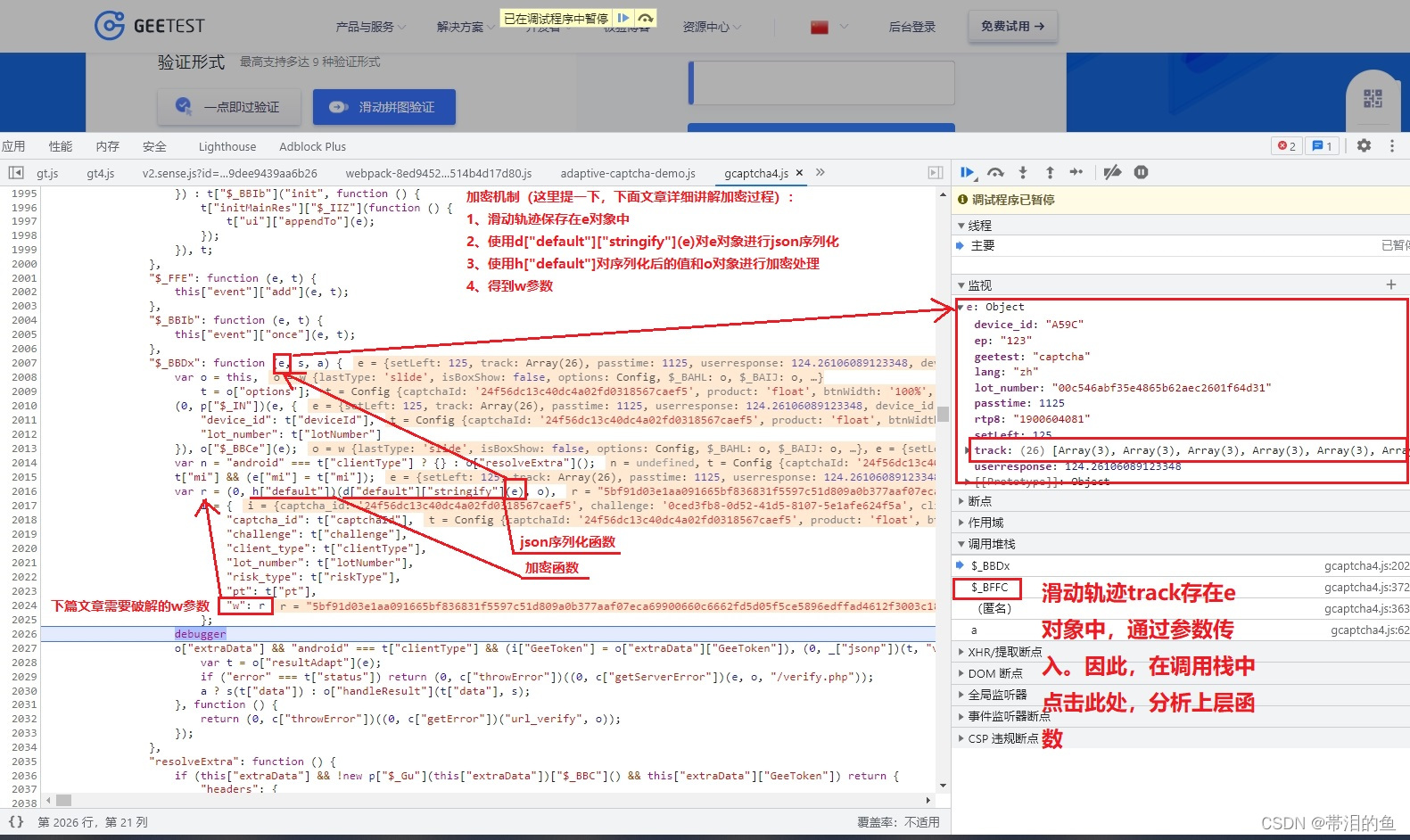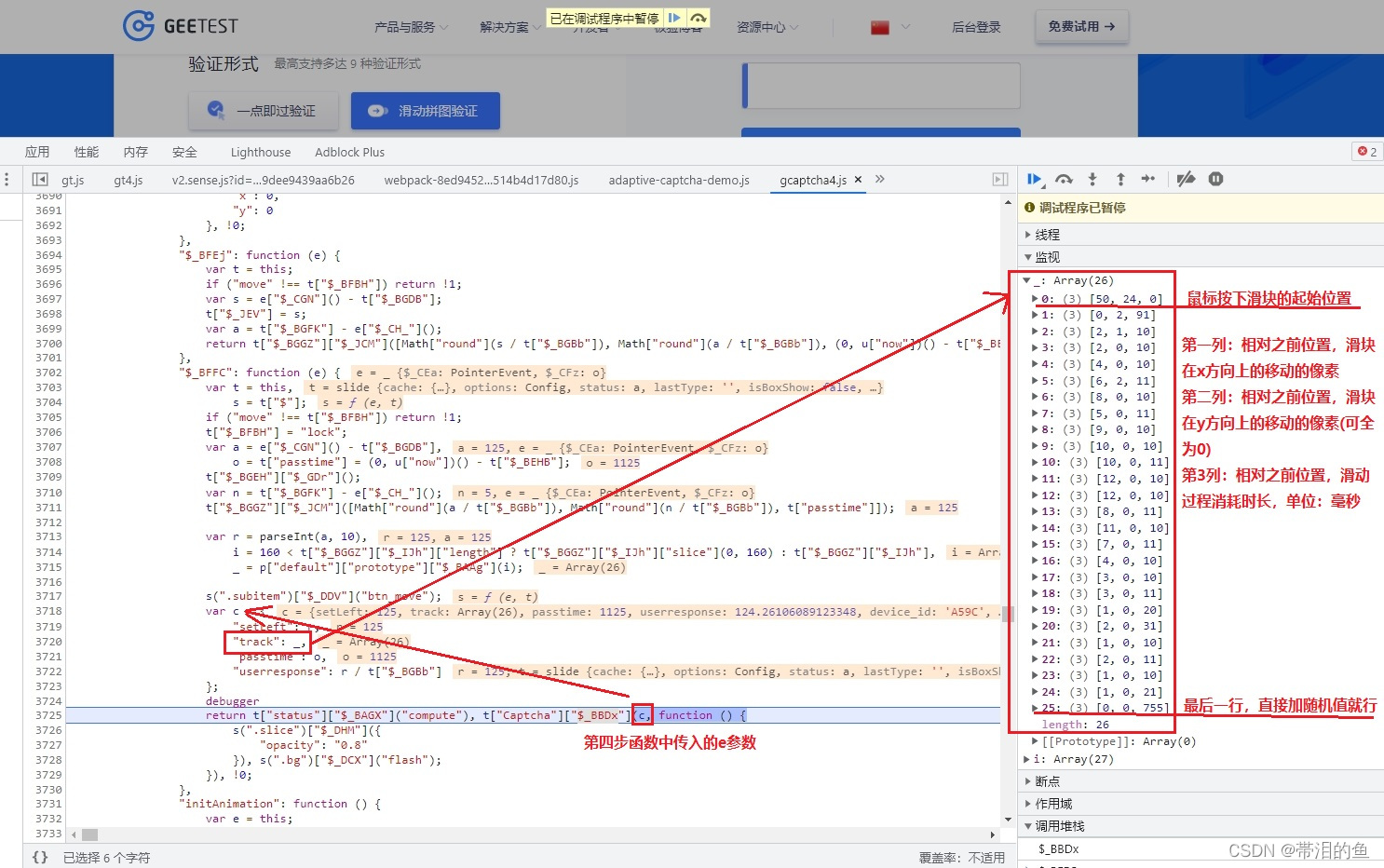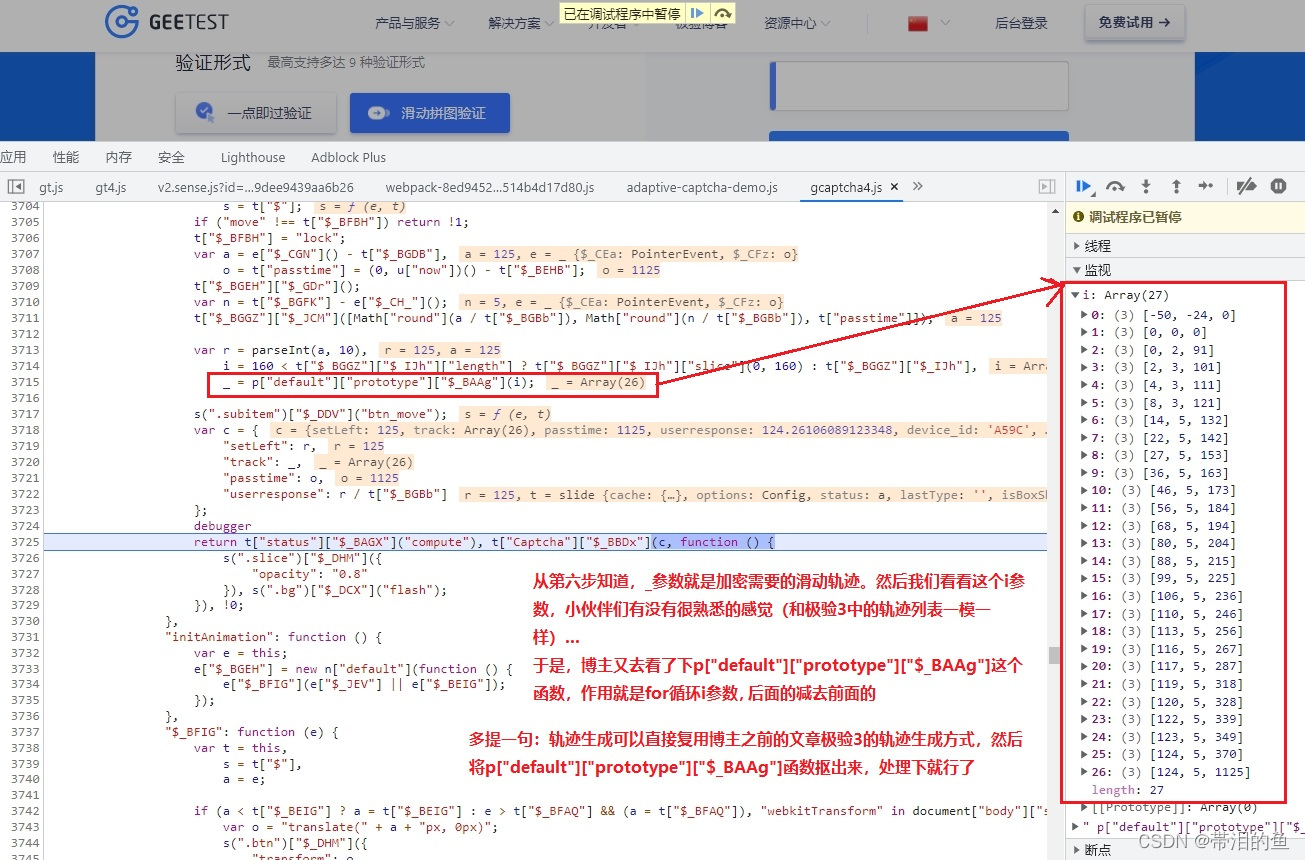# 极验第四代滑块验证码破解（三）：滑块轨迹构造

1. 生成滑动轨迹的js入口• 第六步：多滑动几次，观察滑动轨迹规律，便于自己构造

• 最后：这里我要吐槽下了，极验的研发太不上心了，看看下面的图片就知道了2. 滑动轨迹的python实现

# -*- coding: utf-8 -*-
import random

def __ease_out_expo(x):
if x == 1:
return 1
else:
return 1 - pow(2, -10 * x)

def __ease_out_quart(x):
return 1 - pow(1 - x, 4)

def get_slide_track(distance):
"""
根据滑动距离生成滑动轨迹
:param distance: 需要滑动的距离
:return: 滑动轨迹<type 'list'>: [[x,y,t], ...]
x: 已滑动的横向距离
y: 已滑动的纵向距离, 除起点外, 均为0
t: 滑动过程消耗的时间, 单位: 毫秒
"""

if not isinstance(distance, int) or distance < 0:
raise ValueError(f"distance类型必须是大于等于0的整数: distance: {distance}, type: {type(distance)}")
# 初始化轨迹列表
slide_track = [
[random.randint(20, 60), random.randint(10, 40), 0]
]
# 共记录count次滑块位置信息
count = 30 + int(distance / 2)
# 初始化滑动时间
t = random.randint(50, 100)
# 记录上一次滑动的距离
_x = 0
_y = 0
for i in range(count):
# 已滑动的横向距离
x = round(__ease_out_expo(i / count) * distance)
# 滑动过程消耗的时间
t = random.randint(10, 20)
if x == _x:
continue
slide_track.append([x - _x, _y, t])
_x = x
slide_track.append([0, 0, random.randint(200, 300)])
return slide_track

if __name__ == '__main__':
for _ in get_slide_track(100):
print(_)